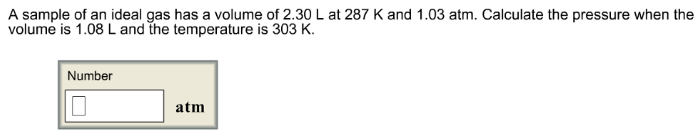# Problem: A sample of an ideal gas has a volume of 2.30 L at 287 K and 1.03 atm. Calculate the pressure when the volume is 1.08 L and the temperature is 303 K.

###### FREE Expert Solution
92% (58 ratings)###### Problem Details

A sample of an ideal gas has a volume of 2.30 L at 287 K and 1.03 atm. Calculate the pressure when the volume is 1.08 L and the temperature is 303 K.Frequently Asked Questions

What scientific concept do you need to know in order to solve this problem?

Our tutors have indicated that to solve this problem you will need to apply the Chemistry Gas Laws: Combined Gas Law concept. You can view video lessons to learn Chemistry Gas Laws: Combined Gas Law. Or if you need more Chemistry Gas Laws: Combined Gas Law practice, you can also practice Chemistry Gas Laws: Combined Gas Law practice problems.